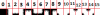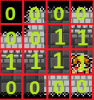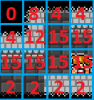# Legacy GMOptimizing a Fluctuating Grid Algorithm

##### Member
Hey all!

Currently I have a system I'm using for "fog of war" or "vision" in my game. Here is what it looks like:I have a "Vision Grid" where each cell holds a true or false value, stating whether or not it is visible to the player. This is updated only when needed, and is pretty much as optimized as I can get it.

The second stage is where I'm stumped as to how to optimize things.
I have a "Shadow Grid" where I hold which Shadow image I need to display. This is generated based on the values found in the Vision Grid of the surrounding tiles.

There are 16 Shadow images, used for the sides, corners, opposing corners, ect.So to help visualize the system a bit, here is a display of each grid, the Vision Grid and then the Shadow Grid:What seems to be the slowdown is calculating the Shadow Grid. Each cell that is visible checks the 8 adjacent cells. If they are not visible, it calculates which Shadow image should be shown.

Now, my math tells me there are 1000s of possible combinations, but I could be wrong on that...So here is the code I'm currently using:

Code:
``````// Cycle Through the whole Grid
for( xx = 0 ; xx < max_x ; xx += 1 )
{
for( yy = 0 ; yy < max_y ; yy += 1 )
{

// If We find a cell the player can see, enter it
if( Grid_Vision[ xx , yy ] = 1 )
{

// Set it so no shadow is shown
Grid_Shade[ xx , yy ] = 15;

// Step through the 8 cells around it
for( new_x = -1 ; new_x < 2 ; new_x += 1 )
{
for( new_y = -1 ; new_y < 2 ; new_y += 1 )
{

// Find which cell we are calculating the image for
// Lets ignore/skip cells that are outside the Grid/Room
if( shadow_x > ( max_x - 2 )){ shadow_x = ( max_x - 2 )}
if( shadow_y > ( max_y - 2 )){ shadow_y = ( max_y - 2 )}

// Make sure the cell we are checking for is not visible
{

// Step through adacent cells from the right in a counter-clockwise order

if( Grid_Vision[ shadow_x + 1 , shadow_y ] = 1 ){ image = 1 }

if( Grid_Vision[ shadow_x + 1 , shadow_y - 1 ] = 1 and image = 0 ){ image = 5 }

if( Grid_Vision[ shadow_x , shadow_y - 1 ] = 1 and image = 0 ){ image = 2 }
if( Grid_Vision[ shadow_x , shadow_y - 1 ] = 1 and image = 1 ){ image = 9 }
if( Grid_Vision[ shadow_x , shadow_y - 1 ] = 1 and image = 5 ){ image = 2 }

if( Grid_Vision[ shadow_x - 1 , shadow_y - 1 ] = 1 and image = 0 ){ image = 6 }
if( Grid_Vision[ shadow_x - 1 , shadow_y - 1 ] = 1 and image = 1 ){ image = 9 }
if( Grid_Vision[ shadow_x - 1 , shadow_y - 1 ] = 1 and image = 5 ){ image = 2 }

if( Grid_Vision[ shadow_x - 1 , shadow_y ] = 1 and image = 0 ){ image = 3 }
if( Grid_Vision[ shadow_x - 1 , shadow_y ] = 1 and image = 1 ){ image = 15 }
if( Grid_Vision[ shadow_x - 1 , shadow_y ] = 1 and image = 2 ){ image = 10 }
if( Grid_Vision[ shadow_x - 1 , shadow_y ] = 1 and image = 5 ){ image = 10 }
if( Grid_Vision[ shadow_x - 1 , shadow_y ] = 1 and image = 6 ){ image = 3 }
if( Grid_Vision[ shadow_x - 1 , shadow_y ] = 1 and image = 9 ){ image = 15 }

if( Grid_Vision[ shadow_x - 1 , shadow_y + 1 ] = 1 and image = 0 ){ image = 7 }
if( Grid_Vision[ shadow_x - 1 , shadow_y + 1 ] = 1 and image = 1 ){ image = 12 }
if( Grid_Vision[ shadow_x - 1 , shadow_y + 1 ] = 1 and image = 2 ){ image = 10 }
if( Grid_Vision[ shadow_x - 1 , shadow_y + 1 ] = 1 and image = 5 ){ image = 14 }
if( Grid_Vision[ shadow_x - 1 , shadow_y + 1 ] = 1 and image = 6 ){ image = 3 }
if( Grid_Vision[ shadow_x - 1 , shadow_y + 1 ] = 1 and image = 9 ){ image = 15 }

if( Grid_Vision[ shadow_x , shadow_y + 1 ] = 1 and image = 0 ){ image = 4 }
if( Grid_Vision[ shadow_x , shadow_y + 1 ] = 1 and image = 1 ){ image = 12 }
if( Grid_Vision[ shadow_x , shadow_y + 1 ] = 1 and image = 2 ){ image = 15 }
if( Grid_Vision[ shadow_x , shadow_y + 1 ] = 1 and image = 3 ){ image = 11 }
if( Grid_Vision[ shadow_x , shadow_y + 1 ] = 1 and image = 5 ){ image = 12 }
if( Grid_Vision[ shadow_x , shadow_y + 1 ] = 1 and image = 6 ){ image = 11 }
if( Grid_Vision[ shadow_x , shadow_y + 1 ] = 1 and image = 7 ){ image = 4 }
if( Grid_Vision[ shadow_x , shadow_y + 1 ] = 1 and image = 9 ){ image = 15 }
if( Grid_Vision[ shadow_x , shadow_y + 1 ] = 1 and image = 10 ){ image = 15 }
if( Grid_Vision[ shadow_x , shadow_y + 1 ] = 1 and image = 14 ){ image = 12 }

if( Grid_Vision[ shadow_x + 1 , shadow_y + 1 ] = 1 and image = 0 ){ image = 8 }
if( Grid_Vision[ shadow_x + 1 , shadow_y + 1 ] = 1 and image = 2 ){ image = 9 }
if( Grid_Vision[ shadow_x + 1 , shadow_y + 1 ] = 1 and image = 3 ){ image = 11 }
if( Grid_Vision[ shadow_x + 1 , shadow_y + 1 ] = 1 and image = 5 ){ image = 1 }
if( Grid_Vision[ shadow_x + 1 , shadow_y + 1 ] = 1 and image = 6 ){ image = 13 }
if( Grid_Vision[ shadow_x + 1 , shadow_y + 1 ] = 1 and image = 7 ){ image = 4 }
if( Grid_Vision[ shadow_x + 1 , shadow_y + 1 ] = 1 and image = 10 ){ image = 15 }
if( Grid_Vision[ shadow_x + 1 , shadow_y + 1 ] = 1 and image = 12 ){ image = 12 }
if( Grid_Vision[ shadow_x + 1 , shadow_y + 1 ] = 1 and image = 14 ){ image = 12 }

}
}
}
}

}
}``````
Basically I run through the Vision Grid looking for cells we can see.
- Then for each of those, if they are not visible to the player, I...
- Find which shadow image to use by checking each of the adjacent 8 cells to that one.

Any thoughts on how this, or any system like it really, could be optimized? Perhaps using a mathamatical equation to reduce or eliminate if statements? Would storing the check value in a var instead of calling the array each time be faster?

Thanks in advance for any input you have!

Last edited:

#### Tthecreator

Alright, let's start by cleaning up that code.
You have a lot of if statements there. You should put those inside a small formula or a few checks.
To do that you have to note a few things:
-tiles that have a shadow on the left, right, top or bottom won't have any corner pieces at all. This means that if you first check all the normal directions, you won't even have to check to corners.
-make a binary tree on paper. A binary tree is a kind of data structure/diagram in which you start at a single node. At that node you make a yes/no true/false on/off decision (hence the name), bringing you to the next node. This is important to visualize what kind of check you'll have to make. Once you make such a diagram you might even be able to make a formulaA small example of what you'd have is:
One of the rules I concluded from this is that if two opposite tiles are dark, the current tile will also become dark.

Now for the formula, leaving the corner pieces out for simplicity: (this will require you to reorder your sprite)
Code:
``````//earlier checks you'll have to write
var isvertical;//have some checks to check if the top and bottom tiles are visible(0), the top tile is dark(1), or the bottom tile is dark(2).
var ishorizontal;//same as vertical but this time with left and right.
if isvertical=0 and ishorizontal=0 then{
//there are cornerpieces
var image = 10 + other_things_you'll_have_to_code.
}else{
//no cornerpieces
var image = isvertical*3+ishorizontal;
}``````

P.S. one other thing I noted is that when you have a precomputed tile to the top or left of your current tile, you don't need to check the left-top tile, since it's already checked by the other tiles to the left and right. I however, didn't know how to implement this in any useful way, but am still leaving it here in case you or someone else does.

##### Member
Wow, thanks for the quick reply!

- Checking the sides first (ignoring the corners) seems like a great way to cut down on processing time.
- Your binary tree tactic makes sense. In a way, I think it is similar to what I already have. But nesting the if statements like you mentioned would cut down processing time alot! Thanks for the pointer.
- Your conclusion that if opposing tiles are dark, this tile will be dark, is spot on! The only exception being for opposing corners ( see images 13 and 14 ). This is only possible when there are two or more Vision sources, which I didn't demonstrate in my example, so my bad! But applying that rule to the rest of the checks would be great!
- I'm super interested in your algorithm there! That was exactly the kind of thing I was hoping for; something that could reduce the if statements alot. I'm still trying to wrap my head around it, but I'll try playing with it over the next couple of days.

#### HammerOn

##### Member
If can you think in 3D lighting techniques/concepts.
Wikipedia said:
ambient occlusion is a shading and rendering technique used to calculate how exposed each point in a scene is to ambient lighting.
In your case, this translates into creating "occlusion grid" of the size of the room and precalculating how much "shadows" a tile can have. For example, everything surrounded in 8-directions by another tile will always by 100% occluded so it values is 0. Corner tiles (like the tile 1x1 in the screenshots) can only have shadow tiles 0, 1, 4, 12 of the 15 types.
Use bitwise operations to store the possibilities in the occlusion grid. The corner tiles, for example, would be "value = (0 | 1 | 4 | 12)". Then, "if (value & 1 << 4)" test for shadow tile 4. "if (value & 1 << 12)" test for shadow tile 12 and so on. In other words, a "shadow map".
This way when calculation the vision grid, you only process what is needed to check and skip everything that isn't even possible.

Also, use something like ds_grid_set_grid_region to copy the region of the occlusion grid around the player to a small grid which is the size the player's field of view. This way you only loop through a few tiles instead of the entire room.

P.S. one other thing I noted is that when you have a precomputed tile to the top or left of your current tile, you don't need to check the left-top tile, since it's already checked by the other tiles to the left and right. I however, didn't know how to implement this in any useful way, but am still leaving it here in case you or someone else does.
This technique is more or less about it but you will know what checks to avoid from the start instead of in real-time.

Last edited:

##### Member

After taking some time to work on things, I thought I would share some results. Maybe one day they might assist someone else?
To make sure I knew which part of the code was taking up the most time, I broke it into several scripts, and ran the profiler.

--- Thanks to Tthecreator's observations, I was able to reduce the computation of the actual shadow image to 15% of the original time!
I used the binary tree for this method.

I tried using the algorithm method too! I never got it to cover every possible situation, so it can't be implemented at the moment. Interestingly, my tests indicate it will run at roughly 10% of the original, so it is better than a binary tree, but not enough for me to pour more time into it right now!CONCLUSION: To compute the image needed, binary tree is substantially faster without being overly complicated to implement!

The surprise to me came when I found out that the actual major slow down wasn't here, it was that the script was running this throughout the room ( 50 x 50 Grid ). This doesn't seem like a lot, but in reality, it was checking each cell, and if visible, then it would check the adjacent ( 8 ) cells, and if not visible or already have a set image, it would calculate it. The game is designed to have up to three points of vision in the room, and level design varies a lot, so there could be some open areas. In my test there were about ( 450 ) cells visible in the level. That means ( 3600 ) calls!

Now, this would only have run every 5 frames or so, when something changed/moved, but it was still too much.

--- This is where HammerOn's input really helped. I wasn't able to use his occlusion grid suggestion, but his observation about only doing what is on the screen was perfect. It's a basic programming principle, but I had questions on whether it would be best to handle that larger load every few frames, or a smaller one every frame. Turns out the latter is much better, because I was able to change my approach too!

Instead of looking for visible cells and then checking the surrounding cells, it straight up just walks through every cell in the view, and computes the shadow if needed. For comparison, the view is ( 13 x 13 ) cells, so with a buffer of 1 on each edge, we come to ( 15 x 15 ), which means ( 225 ) computations per call, instead of the original ( 3600 )! The best part is that it is independent of what is going on in the level or how many cells are visible, so unexpected lagging isn't a problem. I do need to know which cells are visible throughout the level for certain objects to function properly, but not the actual shadow image, so it works great.

CONCLUSION: Check which cells are visible throughout the entire level, but only calculate which images are needed that are on the screen.

Thanks again for your help with this!•Japster and Snayff

•